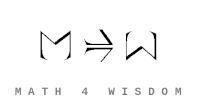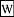文章 发现 ms@ms.lt +370 607 27 665 My work is in the Public Domain for all to share freely. 读物 书 影片 维基百科 Software Upload In what sense are there six natural bases for the symmetric functions? How are the symmetric functions expressing the fundamentals of representation theory, linear algebra and matrix multiplication? How are the symmetric functions expressing the fundamentals of group theory, the symmetric group and permutations? How are the symmetric functions relating automorphisms of lists and automorphisms of sets? Symmetric functions Intuitively understand the Schur functions as the characters of the irreducible representations of the general linear groups. Discover a more natural way to express the Schur functions than the rim hook tableaux. Do this for both symmetric function and for the functions of the eigenvalues. Understand the relations of the Schur functions to the binomial theorem. Consider the Schur functions in terms of the Jacobi-Trudi formulas - and what it means for the eigenvalue case. Understand how the role of the Schur functions and rim hook tableaux depends on the characteristic k of the field. Understand the combinatorics underlying the map between elementary (< decreasing slack) and homogeneous (<= increasing slack) bases, especially as it works in taking the power (=) basis to define the Schur (< x <=) bases. Consider this also in the case of the eigenvalues of a matrix. We also have a foursome, perhaps: Schur - monomial - forgotten? - power. The human bases - monomial and forgotten - map to elementary and homogeneous? Do the six natural bases of the symmetric functions correspond to the six transformations? Understand the elementary symmetric functions in terms of the wedge product. And the homogeneous symmetric functions in terms of the inner product? In category theory, where do symmetric functions come up? What are eigenvalues understood as? What would be symmetric functions of eigenvalues? Symmetric functions of the eigenvalues of a matrix Make sense combinatorially of the map between the homogeneous functions of eigenvalues in terms of words and in terms of products of Lyndon words. How can symmetric functions of eigenvalues model my findings about the combinatorics of orthogonal polynomials? How do symmetric functions of eigenvalues expand on what the trace (the sum of the eigenvalues) says about representations? Sources My Ph.D. thesis: Symmetric Functions of the Eigenvalues of a Matrix John Baez. Higher-Dimensional Algebra II:2-Hilbert Spaces relates adjunctions, Young diagrams, Lie groups John Baez, Todd Trimble. Schur functors Corelations in Network Theory mentions David Ellerman's work that shows that sets-relations are dual to partitions-corelations. Amritanshu Prasad, author of Representation Theory: A Combinatorial Viewpoint Generalized Bernstein Polynomials and Symmetric Functions, Boyer and Thiel, about Bernstein polynomials and Pascal's triangle.Schur functorFirst and second fundamental theorems of invariant theory https://www.symmetricfunctions.com Emily Braley. Eigencone problems for odd and even orthogonal groups. Can relate to symmetric functions of eigenvalues. Notes Relate the monomial, forgotten, Schur symmetric functions of eigenvalues with the matrices {$(I-A)^{-1}$} and {$e^A$}. Exercise: Get the eigenvalues for a generic matrix: 2x2, 3x3, etc. Exercise: Look for a method to find the eigenvalues for a generic matrix. Express the solving of the equation as a way of relating the elementary functions. Are they related to the inverse Kostka matrix? And the impossibility of a combinatorial solution? And the nondeterminism issue, P vs NP? Think again about the combinatorial intepretation of {$K^{-1}K=I$}. What do the constraints on Lie groups and Lie algebras say about symmetric functions of eigenvalues. Vandermonde determinant shows invertible - basis for finite Fourier transform What is the connection between the universal grammar for games and the symmetric functions of the eigenvalues of a matrix? How do symmetries of paths relate to symmetries of young diagrams Partitions, duality, tableaux David Ellerman --- Four New Partition-related Theories. David Ellerman --- The Logical Theory of Canonical Maps. Ellerman thinks in terms of elements and distinctions. To what extent do these express the difference between internal structure and external relationships? An element is related to its set, as unequals, whereas distinctions relate equals. This is also the difference between a tree-structure and a network-structure. And a sequence distills and/or (re-)introduces the distinction between "from" and "to". David Ellerman at Azimuth Forum Kostka matrix Symmetric functions of eigenvalues Think of the roots of a polynomial (and the eigenvalues of a matrix) as inverting it.Adams operation relates symmetric functions to K-theory. How is the Schur functor (and its direct sums and tensor products) related to the Young diagrams? And to Schur-Weyl duality? And what do the six bases look like? And how does it relate to the symmetric functions of the eigenvalues of a matrix?
Šis puslapis paskutinį kartą keistas February 23, 2022, at 03:18 PM